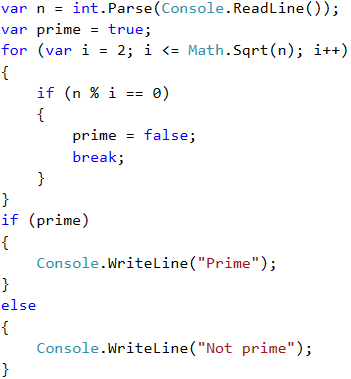# Example: Prime Number Checking

The next problem we are going to solve is to check whether given number is prime. An integer is prime if it cannot be decomposed to a product of other numbers. For example: 2, 5 and 19 are primes, while 9, 12 and 35 are composite.

## Video: Prime Number Checking

Watch this video lesson to learn how to check if a number is prime: https://youtu.be/4lWOaPWKf0I.

## Hints and Guidelines

Before proceeding to the hints about solving the "prime checking" problem, let's recall in bigger detail what prime numbers are.

Definition: an integer is prime if it is divisible only by itself and by 1. By definition, the prime numbers are positive and greater than 1. The smallest prime number is 2.

We can assume that an integer n is a prime number if n > 1 and n is not divisible by a number between 2 and n-1.

The first few prime numbers are: 2, 3, 5, 7, 11, 13, 17, 19, 23, 29, 31, 37, 41, 43, …

Unlike the prime numbers, composite numbers are integers which can be obtained by multiplying several prime numbers.

Here are some examples of composite numbers:

• 10 = 2 * 5
• 42 = 2 * 3 * 7
• 143 = 13 * 11

Positive integers, greater than 1, can be either prime or composite (product of primes). Numbers like 0 and 1 are not prime, but are also not composite.

We can check if an integer is prime following the definition: check if n > 1 and n is divisible by 2, 3, …, n-1 without reminder.

• If it is divisible by any of the numbers, it is composite.
• If it is not divisible by any of the numbers, then it is prime.We can optimize the algorithm instead of checking it to n-1, to check divisors to √n. Think what the reason for that is!

## Prime Checking Algorithm

The most popular algorithm to check if a number n is prime is by checking if n is divisible by the numbers between 2 and √n.

The steps of the "prime checking algorithm" are given below in bigger detail:

• We create the variable n to which we assign an integer taken from the console input.
• We create an isPrime bool variable with an initial value true. We assume that a number is prime until proven otherwise.
• We create a for loop in which we set an initial value 2 for the loop variable, for condition the current value <= √n. The loop step is 1.
• In the body of the loop, we check if n, divided by the current value, has a remainder. If there is no reminder from the division, then we change isPrime to false and we exit the loop through the break operator.
• Depending on the value of isPrime, we print whether the number is prime (true) or composite (false).

## Implementation of the Prime Checking Algorithm

Here is a sample implementation of the prime checking algorithm, described above:What remains is to add a condition that checks if the input number is greater than 1, because by definition numbers such as 0, 1, -1 and -2 are not prime.